# Newton's Laws of Motion

While Newton’s laws of motion may seem obvious to us today, centuries ago they were considered revolutionary. The three laws of motion help us understand how objects behave when they are standing still, when moving and when forces act upon them. This article is a description of Sir Isaac Newton’s Laws of motion and a summary of what they mean.

## Newton’s First Law of Motion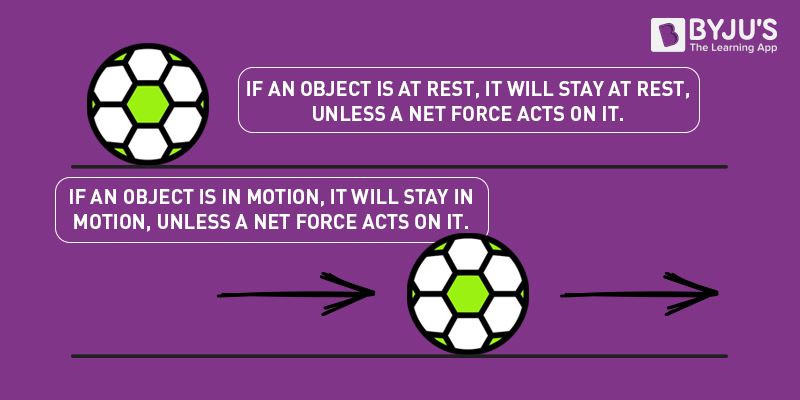The first law of motion implies that things cannot start, stop, or change direction all by themselves. It requires some force from the outside to cause such a change. This property of massive bodies to resist changes in their state of motion is called inertia. Newton’s first law is also known as the law of inertia.

Newton’s 1st law states that a body at rest or uniform motion will continue to be at rest or uniform motion until and unless a net external force acts on it.

The crucial point here is that if there is no net force resulting from unbalanced forces acting on an object, then the object will maintain a constant velocity. If that velocity is zero, then the object remains at rest. And if an additional external force is applied, the velocity will change because of the force.

Read More: Newton’s First Law of Motion

## Newton’s Second Law of Motion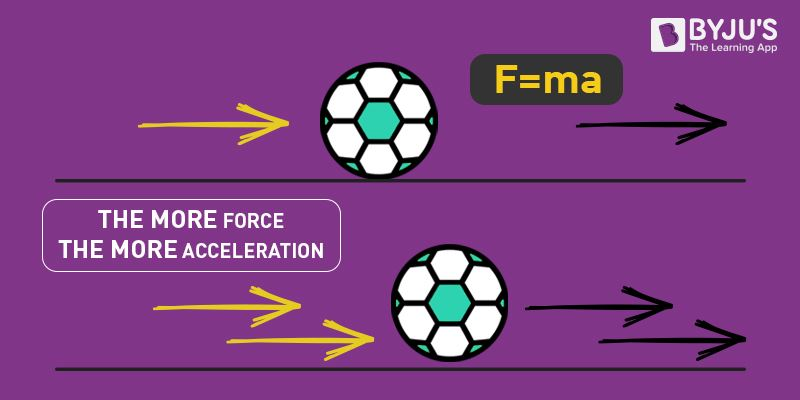The second law of motion describes what happens to the massive body when acted upon by an external force. The 2nd law of motion states that the force acting on the body is equal to the product of its mass and acceleration.

Newton’s 2nd law states that the acceleration of an object as produced by a net force is directly proportional to the magnitude of the net force, in the same direction as the net force, and inversely proportional to the mass of the object.

Newton’s second law describes precisely how much an object will accelerate for a given net force.

Mathematically, we express the second law of motion as follows:

$f\propto \frac{dP}{dt}\\ \\ \Rightarrow f\propto \frac{mv-mu}{t}\\ \\ \Rightarrow f\propto\frac{m(v-u)}{t}\\ \\ \Rightarrow f\: \propto \: ma\\ \\ \Rightarrow f=k\, ma$

In the equation, k is the constant of proportionality, and it is equal to 1 when the values are taken in SI unit. Hence, the final expression will be,

$F=ma$

Read More: Newton’s Second Law of Motion

## Newton’s Third Law of Motion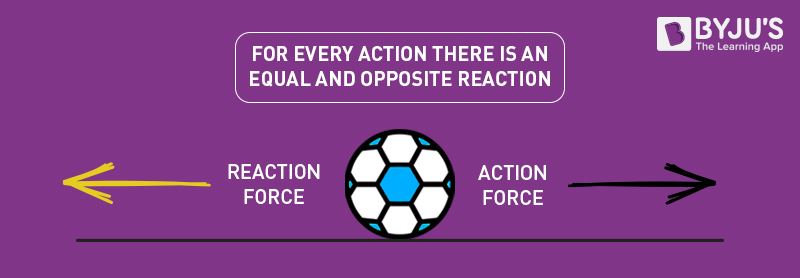The third law of motion describes what happens to the body when it exerts a force on another body.

The Newton’s 3rd law states that for every action there is an equal and opposite reaction.

When two bodies interact, they apply force on each other that are equal in magnitude and opposite in direction. To understand Newton’s third law with the help of an example, let us consider a book resting on a table. The book applies a downward force equal to its weight on the table. According to the third law of motion, the table applies an equal and opposite force on the book. This force occurs because the book slightly deforms the table; as a result, the table pushes back on the book like a coiled spring. Newton’s third law of motion implies the conservation of momentum.

Read More: Newton’s Third Law of Motion

## Review Questions

1) While driving to work, Naveen always keeps his purse on the passenger seat.  By the time he gets to work, his purse would have fallen on the floor in front of the passenger seat. One day, he asks you to explain why this happens in terms of physics. What do you say?

We can explain this to Naveen in terms of Newton’s first law of motion. The purse due to inertia travels forward with the car until acted upon by another force, in this case, the force of the car floor.

2) Which of Newton’s laws best explains how a magician can pull a tablecloth from underneath dishes?
Newton’s first law of motion can explain how a magician pulls a tablecloth from underneath the dishes. A negligible horizontal force is applied during the process. As per Newton’s first law of motion, the dishes and glasses remain in their state of motion (rest); as a result, they remain undisturbed. The table cloth in the trick is made so slippery such that it doesn’t apply any frictional force on glasses and dishes.

### Understanding laws of motion with engaging video lecture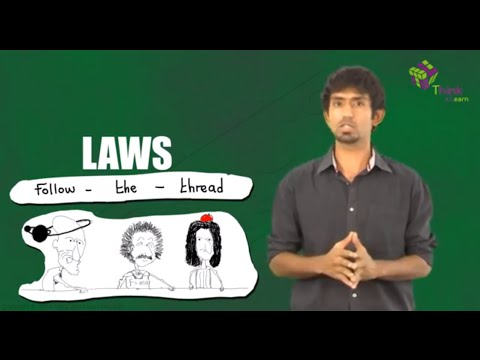## Laws of Motion Summary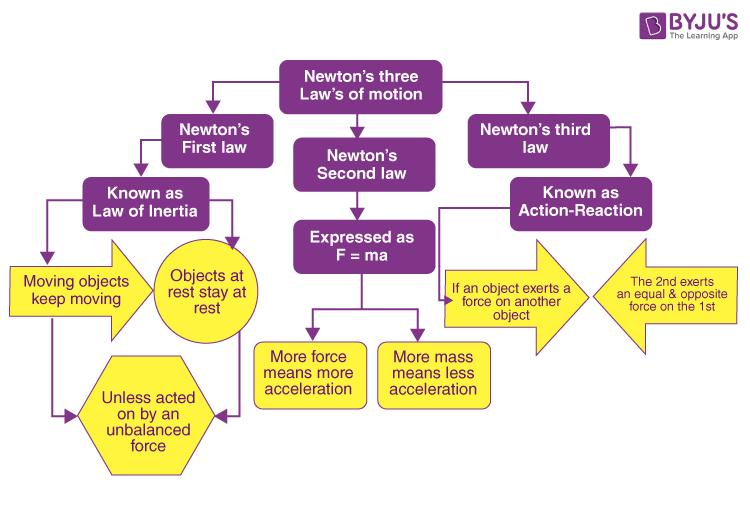## Laws of Motion Problems with Solution

### 1. Suppose a bike with a rider on it having a total mass of 63 kg brakes and reduces its velocity from 8.5 m/s to 0 m/s in 3.0 second. What is the magnitude of the braking force?

Solution:

The combined mass of the rider and the bike = 63 kg
Initial Velocity = 8.5 m/s
Final Velocity = 0 m/s
The time in which the bike stops = 3 s

The net force acting on the body is equal to the rate of change of momentum of the object.

$F=\frac{\Delta p}{\Delta t}$

The momentum of a body with mass m and velocity v is given by  p = mv

Hence, the change in momentum of the car is given by

$\Delta p=mv-mu=m(v-u)$

Hence, the net force acting on the car is given by

$F=\frac{m(v-u)}{\Delta t}$

Substituting the value, we get

$F=\frac{63\,kg\times (0-8.5\,m/s)}{3.0\,s}=1.8\times 10^2\,N$

$F=1.8\times 10^2\,N$

### 2. Calculate the net force required to give an automobile of mass 1600 kg an acceleration of 4.5 m/s2

We calculate the force using the following formula

$F=ma$

Substituting the values in the equation, we get

$F=100\,kg\times 4.5\,m/s^2=7200\,N$

$F=7200\,N$

## Frequently Asked Questions – FAQs

### Who discovered the three laws of motion?

Sir Isaac Newton discovered the three laws of motion.

### Why are the laws of motion important?

Newton’s laws are essential because they relate to everything that we do or see in everyday life. These laws tell us how things move or stay still, why we don’t float out of our bed or fall through the floor of our house.

### What is Newton’s laws of motion all about?

Newton’s laws of motion imply the relationship between an object’s motion and the forces acting on it. In the first law, we come to understand that an object will not change its motion unless a force acts on it. The second law states that the force on an object is equal to its mass times its acceleration. And, finally, the third law states that for every action, there is an equal and opposite reaction.

### What is the difference between Newton’s laws of motion and Kepler’s laws of motion?

Newton’s laws of motion are general and apply to any motion, while Kepler’s laws of motion apply only to planetary motion in the solar system.

### What are some daily life examples of Newton’s 1st, 2nd and 3rd laws of motion?

• The motion of a ball falling through the atmosphere, or a model rocket being launched up into the atmosphere are both excellent examples of Newton’s 1st law.
• Riding a bicycle is a good example of Newton’s 2nd law. In this example, the bicycle is the mass. The leg muscles pushing on the pedals of the bicycle is the force.

• You hit a wall with a certain amount of force, and the wall returns that same amount of force. This is an example of Newton’s 3rd law.

Stay tuned to BYJU’S and Fall in Love with Learning!

Test your knowledge on Laws of motion

#### 28 Comments

1. siddharth singh baghel

it is very helpful and useful i want to thank BY JU’S

2. Nouseen

Super

3. neha bhange

Good enough

1. prathmesh shewale

nice

2. prathmesh shewale

good

4. Thanks for the information

5. mohd mujeeb

Very good super

6. Kruthika. B

Excellent

7. Angela Sherpa

Good notes

8. really helpful, thanks!

9. Aishwarya

Good information!

10. Man suryawanshi

Nice exaplaination

11. narmadha ravi

The concepts are clear and easy to understand and to study. so, i feel comfortable with this app .

12. Good article

13. Pranjal Borkar

Good explanation of concept.

14. Good

15. Anantya

Thanks
This really helped me😀
I will secure 🥇🏆

16. Md Mohsin

Super Explanation

17. Madhumitha Devaraj

Good to learn

18. Shweta Singh

This article cleared all my doubts about the laws of motion.
Thanks, BYJU’S.

19. Satyarth Khajuria

The best content for visualization and it cleared all my doubts. I personally think that BYJU’S is the best app in terms of e-learning. Thanks to BYJU’S

20. Monica

Neat and Crisp Content!

21. Vishwateja

Wow! Super and very useful content provided here.

22. Dhruvitha

A very useful content for making notes

23. Shehzad Ahmed

Nice one

24. Thank you so much you helped in my project I ended up citing almost all my work from your website😅

25. Nice

26. It was nice 🙂